# SQL SERVER Aggregate Windows Functions with Examples

Database users are often required to calculate the Average or SUM of the total transaction done by the Customer or User in real-time scenarios. SQL Server Aggregate functions make the life of the developer easier to calculate SUM, AVERAGE, COUNT, MAXIMUM, and MINIMUM from the transactions done by the given Customer or User.

Aggregate Functions are always used to perform some kind of calculation over one or more values and return a single scalar value.

SQL Server provides the following Aggregate function

• AVG
• SUM
• MAX
• MIN
• COUNT

Windows Functions on the other hand operates on set of rows called a window frame and return a single value for each row from the underlying query. Window is defined using the OVER() Clause and this clause allows  defining a window based on a specific column(similar to Group By clause).

The OVER() Clause has following capabilities

• Defines window partitions to form group of rows using PARTITION BY Clause.
• Order rows within a partition using ORDER BY Clause.﻿﻿

Combining the Aggregate function with windows functions row retain their identity and also show an aggregated value for each row whereas the normal GROUP BY clause combines then hides the individual rows being aggregated in the final result set.

Let me explain the above using an example.

To demonstrate I am using AdventureWorks2014 sample Database and considering the table named Sales.SalesOrderHeader in the Database which has some transaction data.

Number of records in this table

`select Count(*) from Sales.SalesOrderHeader﻿﻿`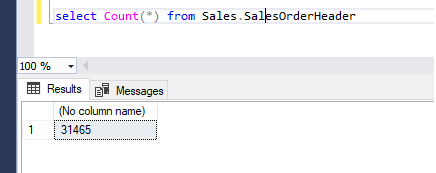For Simplicity We will work on three columns of this table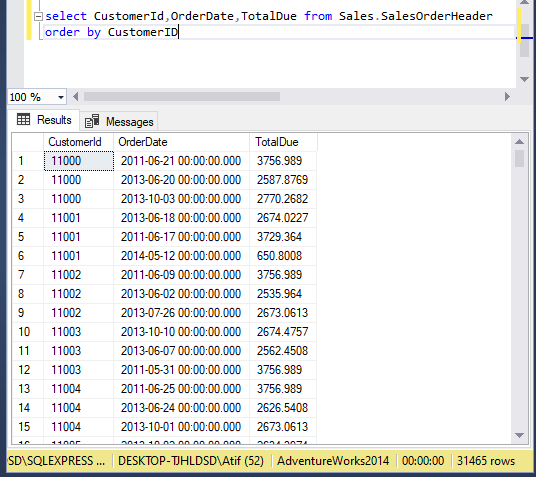As you can see from the above screenshot we have 31465 records in the Sales.SalesOrderHeader table.

If we want to calculate SUM of TotalDue by each customerId using Group By clause then it hides individual rows being aggregated as shown below

`select customerid, SUM(TotalDue) As sum_total_due_per_customer_idfrom Sales.SalesOrderHeader group by CustomerIDorder by CustomerID`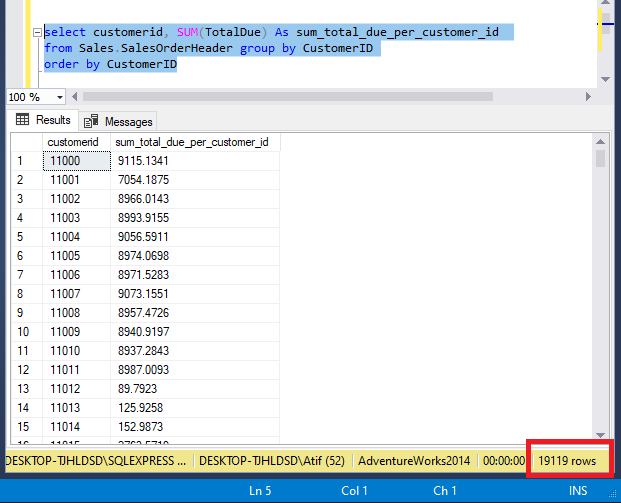Whereas when we calculate SUM of TotalDue by each customer Id using Window Function return all the rows of the original table and doesn't hide the rows being aggregated.

`select customerid,totaldue,SUM(TotalDue) OVER(PARTITION BY CUStomerID) As sum_total_due_per_customer_idfrom Sales.SalesOrderHeader`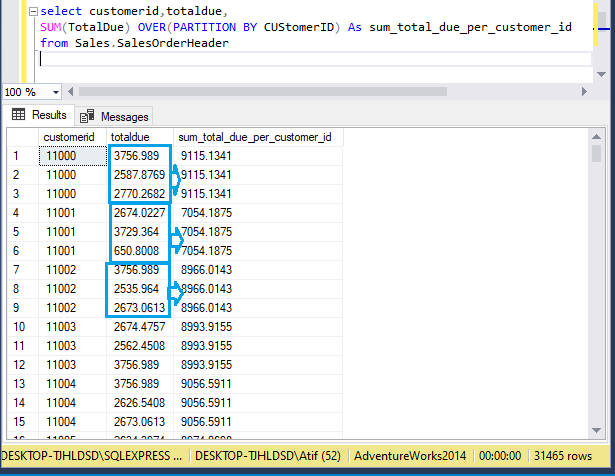Similarly, we can combine the yearly SUM of total dues by each customer

`select customerid,orderdate,totaldue,SUM(TotalDue) OVER(PARTITION BY CUStomerID,year(Orderdate))As sum_total_due_per_customer_id_by_yearfrom Sales.SalesOrderHeader`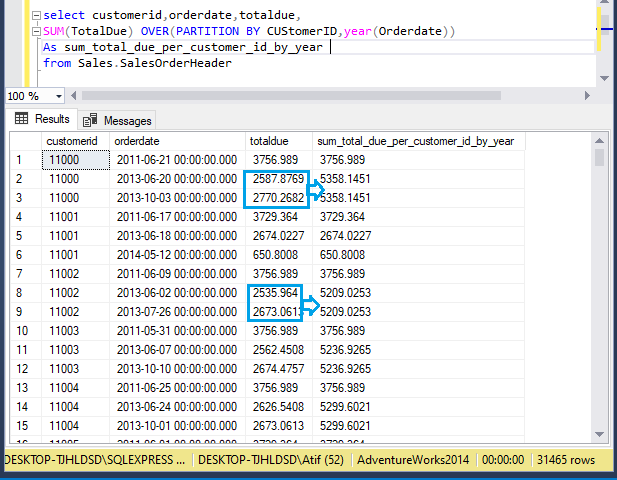As you can see above customerid 11000 has a total of 3 orders and 2 out of 3 orders has order date of the year 2013 so we are calculating the sum of total dues on yearly basis on a given customerid.

Similarly, we can use other Aggregate functions with the Window function to get a similar result.

`SELECT  CustomerID,  OrderDate,  TotalDue,  SUM(TotalDue) OVER(PARTITION BY CustomerID , YEAR(OrderDate)) AS sum_of_total_due_per_year,  AVG(TotalDue) OVER(PARTITION BY CustomerID , YEAR(OrderDate)) AS average_of_total_due_per_year,  MIN(TotalDue) OVER(PARTITION BY CustomerID , YEAR(OrderDate)) AS minimum_of_total_due_per_year,  MAX(TotalDue) OVER(PARTITION BY CustomerID , YEAR(OrderDate)) AS maximum_of_total_due_per_year,  COUNT(*) OVER(PARTITION BY CustomerID , YEAR(OrderDate)) AS count_of_total_due_per_year  FROM Sales.SalesOrderHeader`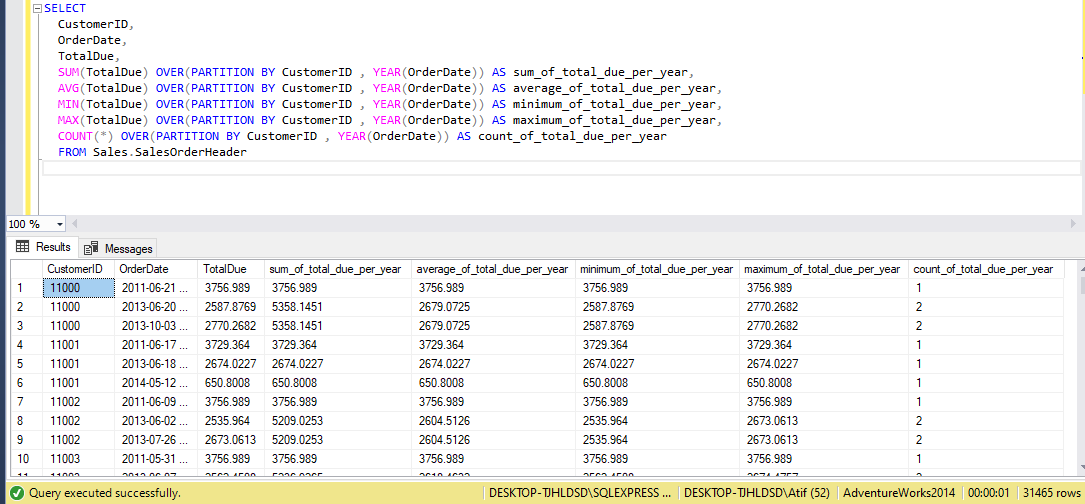Hope you have enjoyed this article and got an understanding regarding the SQL Server Aggregate Windows function.